# Sum of an array using Multithreading in C/C++

In this article, we are going to see how we can use multithreading while summing up an array in C/C++?
Submitted by Radib Kar, on November 05, 2020

In my last article, we saw details about why we need multithreading and how can we use multithreading in C/C++? Here, is a live example for you which will show you the application of multithreading, a real-world program with the proper implementation and also analysis if we had any improvements.

The problem statement is pretty simple.

Sum up an array of 10000000 elements where are elements are consecutive natural numbers

That means it's basically finding the sum of 1 to 1,00,00,000

Firstly we see the naïve program without threading

```#include <stdio.h>
#include <stdlib.h>
#include <time.h>

#define MAX_NO_OF_ELEMENTS 10000000

static long long int sum;
static int arr[MAX_NO_OF_ELEMENTS];

int main()
{
for (int i = 0; i < MAX_NO_OF_ELEMENTS; i++)
arr[i] = i + 1;

clock_t start, end;
double cpu_time_taken;

start = clock();

//summing

for (int i = 0; i < MAX_NO_OF_ELEMENTS; i++)
sum += arr[i];

end = clock();
cpu_time_taken = ((double)(end - start)) / CLOCKS_PER_SEC;

printf("Total sum: %lld\n", sum);

printf("Time taken to sum all the numbers are %lf\n", cpu_time_taken);

return 0;
}
```

Output: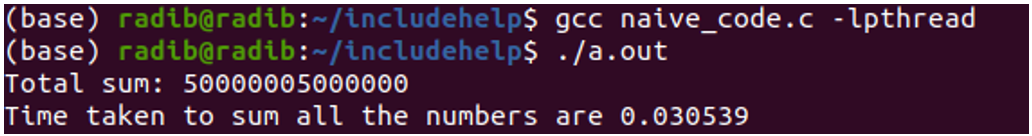Now here we go with the multithreading implementation. Let us first see the code then we will discuss.

```#include <stdio.h>
#include <stdlib.h>

#define MAX_NO_OF_ELEMENTS 10000000

typedef struct arg_data {
} arg_data;

//shared data on which threads will work concurrently
//array which will be summed
static int arr[MAX_NO_OF_ELEMENTS];
//sum variable that will store the total sum
static long long int sum;

void* worker_sum(void* arg)
{

int startpart = endpart - (MAX_NO_OF_ELEMENTS / MAX_NO_OF_THREADS);

printf("Here we will sum %d to %d\n", arr[startpart], arr[endpart - 1]);

long long int current_thread_sum = 0;
for (int i = startpart; i < endpart; i++) {
}

return NULL;
}

int main()
{
//let the array consists of first MAX_NO_OF_ELEMENTS integers,
//1 to MAX_NO_OF_ELEMENTS
for (int i = 0; i < MAX_NO_OF_ELEMENTS; i++)
arr[i] = i + 1;

//argument data to send in worker functions

//total number of threads we will create

clock_t start, end;
double cpu_time_taken;

start = clock();

}

//joining the threads one by one
for (int i = 1; i <= MAX_NO_OF_THREADS; i++)

end = clock();
cpu_time_taken = ((double)(end - start)) / CLOCKS_PER_SEC;

printf("All child threads has finished their works...\n");

printf("Total sum: %lld\n", sum);

printf("Time taken to sum all the numbers are %lf\n", cpu_time_taken);

return 0;
}
```

Since we covered the threading part in our pre-requisite article, I am not bringing those staffs again like how to create a thread, what is thread join and all.

So what we have done here is we created a thread pool with a suitable number of threads. I have created a thread pool with 4 threads. If you have more number of CPU cores, you can increase the number, but in that case, be sure to change the worker function to(to adjust what part a thread is supposed to read)

Now we want that each thread will find the sum of a particular part only.

Since we have 4 threads and total array size is 10000000,

We want that

1st thread will sum 1 to 2500000

2nd thread will sum 2500001 to 5000000

3rd thread will sum 5000001 to 7500000

4th thread will sum 7500001 to 10000000

That's why we have passed the thread number as an argument to the worker function with help of a struct and the range of numbers to sum for a thread is

```endpart=(thread_number)*(MAX_NO_OF_ELEMENTS/MAX_NO_OF_THREADS);
```

This ensures that, though the threads will run concurrently, it will never break the data integrity, as it will never sum the same number multiple times. This has ensured that though the thread work on a shared data resource, they are independent of each other.

Finally, we have joined all the child threads before printing the final sum(total sum)

Below is the output for multiple runs. Now why I have run multiple times that I will cover on the conclusion part of this article.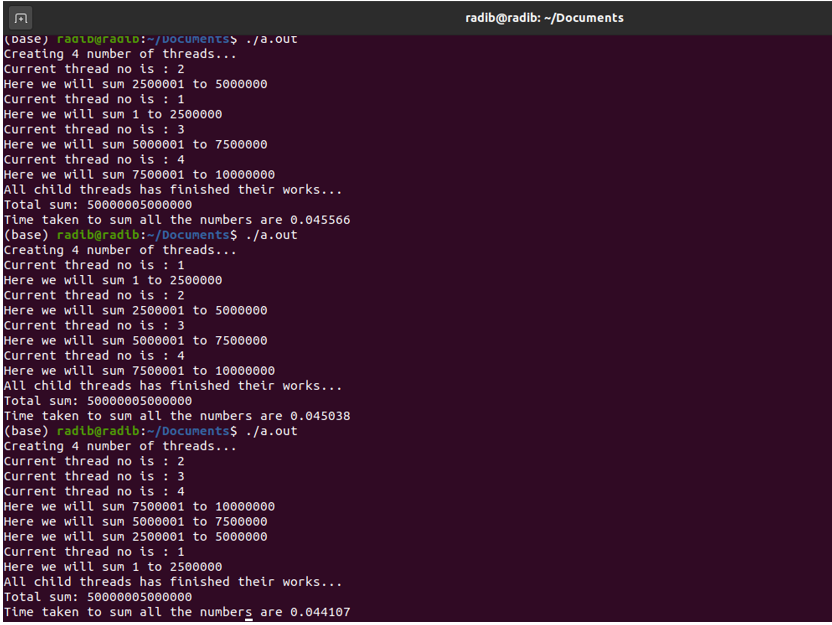Comparison Between the naïve implementation & multithreading implementation

Below is the output of two runs where the first one is the naïve one and the second one is the multithreaded implementation one.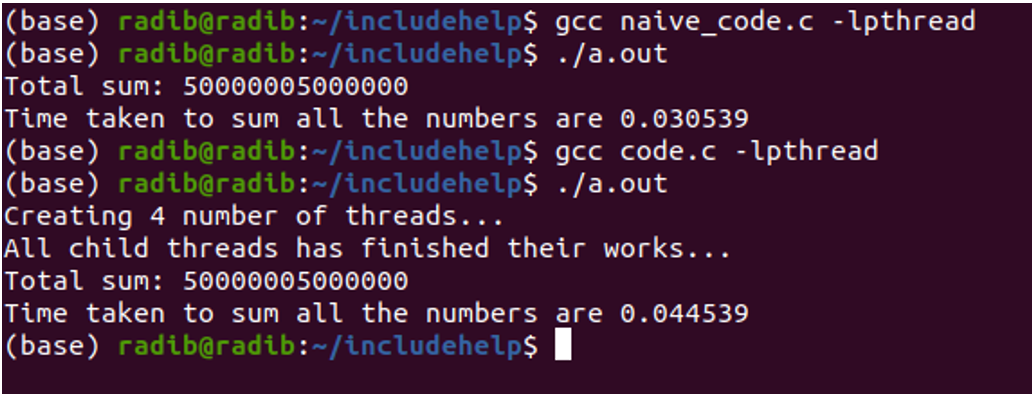While comparing I have commented out the printing additional statements in the worker function as that would take extra time otherwise.

Now, you can see that the naïve run has taken lesser time. So it seems that multithreading is not at all useful as it has taken more time. But that's not the case. Multithreading has additional timing because of the worker function call, thread creation, thread joining etc. Since, here the program was pretty simple and didn't require high computation, function calls, that's why the naïve implementation has taken less time here. But it won't be the case if you do high computations, such as operating on any large data where you don't need execution to happen sequentially. In such cases, multithreading is extremely useful as you can utilize your CPU properly.

Now let's come to the conclusion. Why I have made multiple runs? I have made multiple runs to show you the concurrency. If you see all three outputs minutely, you will find that the order of the printed statements are different which proves that the execution of threads has been different every time. It depends on your OS, how it manages thread execution. But one thing is common is the final result. That is because we have made sure that all child thread will finish their executions before printing the final sum. We are not bothered about the order of thread execution and they are independent, we just want all threads to finish their execution before we print our sum.

The output of run1: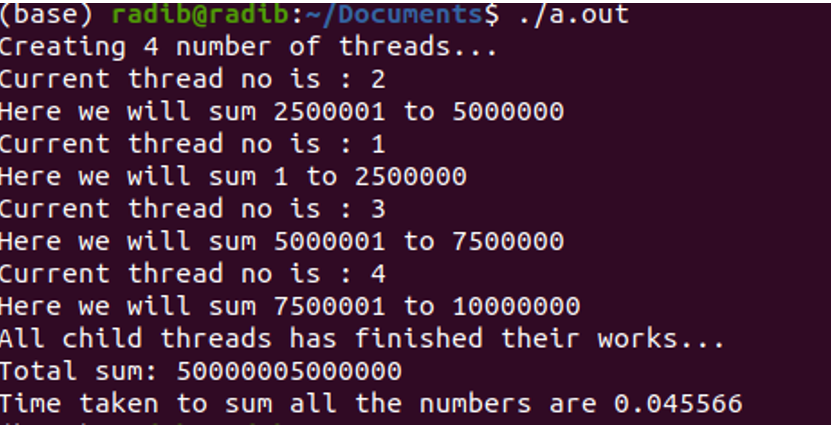Here, thread 2 has been started first, then 1, then 3 and then 4

The output of run2: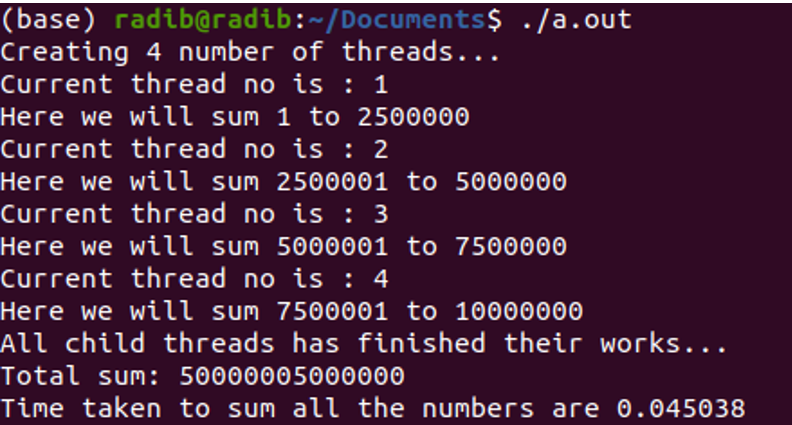Here the thread starting order is 1, 2, 3, 4

The output of run 3: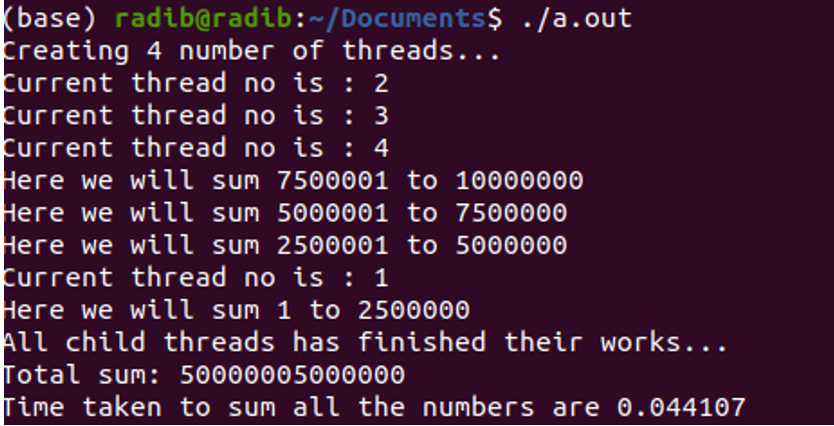Here, you can see that thread 2, 3, 4 has started all together, that's what we say concurrency.